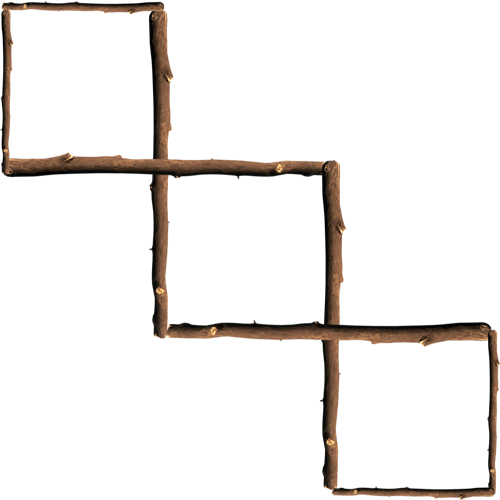A riddle problem is a question or statement intentionally phrased so as to require ingenuity in ascertaining its answer or meaning, typically presented as a game.  Below is a list of the past problem of the week riddles in descending chronological order.

Riddle: Take eight small sticks, four of which are half the length of the other four. How can you make three equal squares out of the sticks?
Answer: Use the longer four sticks to be sharing sides between the squares and at the end their should be three intertwined squares.Riddle: A horse is tied to a five-meter rope in front of an old saloon. Six meters behind the horse is a bale of hay. Without breaking his rope, the horse is able to eat the hay whenever he chooses. How is this possible?
Answer: The rope is not tied to anything else.
Riddle: The ages of a father and son add up to 66. The father's age is the son's age reversed. How old could they be? (3 possible solutions).
Answer: 51 and 15. 42 and 24. 60 and 06.
Riddle: What arithmetic symbol can we place between 2 and 3 to make a number greater than 2 but less than 3?
Riddle: King Tut died 120 years after King Eros was born. Their combined age when they died was 100 years. King Eros died in the year 40 B.C. In what year was King Tut born?
Answer: King Tut was born in 20 B.C. There were 120 years between the birth of King Eros and the death of King Tut, but since their ages amounted to only 100 years, there must have been 20 years when neither existed. This would be a period between the death of King Eros, 40 B.C., and the birth of King Tut, 20 B.C.
Riddle: Jim was examining an angle measuring 14 and 1/2 degrees, using his magnifying glass that magnifies everything two times. Under the glass, how large would that angle measure?
Answer: 14 and 1/2 degrees. Explanation, angles remain constant when magnified. A square has 4-90 degree corners, if you zoom in (magnify) a square, it's still a square.
Riddle: What two whole, positive numbers that have a one-digit answer when multiplied and a two-digit answer when added?